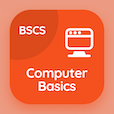Class 10 Online Courses

Grade 10 Physics MCQ PDF - Chapters

# Current Electricity Quiz Questions Online p. 19

Study Current electricity quiz questions and answers, current electricity MCQ questions with answers PDF to solve grade 10 physics quiz 19 for online certification. Potential Difference MCQs, current electricity quiz questions and answers for online classes. "Current Electricity Quiz" PDF Book: potential difference, ohmic and non ohmic conductors, electric power, how does material effect resistance test prep for distance learning classes.

"How much current flows through a body if it touches the terminals of a 12 V battery at 100,000 Ω resistance?" Multiple Choice Questions (MCQ) on current electricity with choices 2.3 × 10-4, 1.2 × 10-4, 2.58 × 10-4, and 4 × 10-4 for online classes. Learn potential difference quiz questions for school certificate programs for online school classes.

## Current Electricity Questions and Answers

MCQ: How much current flows through a body if it touches the terminals of a 12 V battery at 100,000 Ω resistance?

1.2 × 10-4
2.3 × 10-4
2.58 × 10-4
4 × 10-4

MCQ: A temperature-dependent resistor of which resistance decreases as temperature rises are called

voltmeter
thermometer
thermistor
ammeter

MCQ: Over a large range of applied voltages, ohmic conductor have a

linear voltage current relationship
vibratory voltage current relationship
circular voltage current relationship
random voltage current relationship

MCQ: The amount of energy supplied by the current in unit time is called

electric energy
friction
resistance
Electric power

MCQ: The specific resistance of iron is

5.25 × 10-8
1.69 × 10-8
9.8 × 10-8
2.75 × 10-8